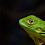## Thursday, May 5, 2016

### Probability is hard

For more than a month, my colleague Sanjoy Mahajan and I have been banging our heads on a series of problems related to conditional probability and Bayesian statistics.  We knew when we started that this material is tricky, as demonstrated by veridical paradoxes like the Monty Hall problem, the Girl Named Florida, and so on.  But even though we were prepared, we have been surprised, continually, by how long it is taking and how effectively we have confused ourselves and each other.

A few times we have hit a brick wall on a hard problem and made a strategic retreat by working on a simpler problem.  At this point, we have retreated all the way to what I'll call the Red Dice problem, which goes like this:
Suppose I have a six-sided die that is red on 2 sides and blue on 4 sides, and another die that's the other way around, red on 4 sides and blue on 2.
I choose a die at random and roll it, and I tell you it came up red. What is the probability that I rolled the second die (red on 4 sides)?  And if I do it again, what's the probability that I get red again?
There are several variations on this problem, with answers that are subtly different.  I explain the variations, and my solution, in a Jupyter notebook:

You can read a static version of the notebook here.

OR

You can run the notebook on Binder.

If you click the Binder link, you should get a home page showing the notebooks and Python modules in the repository for this blog.  Click on red_dice.ipynb to load the notebook for this article.

Once we have settled the Red Dice problem, we will get back to the original problem, which relates to interpreting medical tests (a classic example of Bayesian inference that, again, turns out to be more complicated than we thought).

UPDATE:  After reading the notebook, some readers are annoyed with me because Scenarios C and D are not consistent with the way I posed the question.  I'm sorry if you feel tricked -- that was not the point!  To clarify, Scenarios A and B are legitimate interpretations of the question, as posed, which is deliberately ambiguous.  Scenarios C and D are exploring a different version because it will be useful when we get to the next problem.

1.In this case, you can straightforwardly calculate favorable probability out of total probability, so

[ (1/2).(4/6) ] / [ (1/2).(4/6) + (1/2).(2/6) ]
= [ 4/6 ] / [ (4/6) + (2/6) ]
=  / [2 + 1] = 2/3

But you already knew that. Anyways, it's nice to be able to comment something; most of your posts are way above my paygrade.

1.Good so far!

But I added a followup question: what's the probability that the outcome of the next roll is red, too? Hint: the scenario is deliberately ambiguous.

2.if A is the event that you have the R-favorable die, then as above it's probability P(A) after one roll is now 2/3.
So P(R) = P(R|A)P(A) + P(R|Ac)P(Ac) = (2/3)*(2/3)+(1/3)*(1/3)=5/9

1.That is correct in Scenario B (where I choose a die once and then roll it repeatedly).

3.For what it's worth, I was one of those who were puzzled (I wouldn't say annoyed) by the apparent mismatch between the question as posed and Scenarios C and D.

At the moment, I'm mostly curious about where this is going. All of the calculations in the notebook seem correct to me, and none seem particularly counterintuitive or surprising. I gather that you're building to something surprising, and I look forward to seeing what it is.